• 上篇博文采样sinc函数内插的方式实现了模拟信号的重建：【 MATLABMATLAB 实现模拟信号采样后的重建（一） 这篇博文我们使用零阶保持器（ZOH）来重建信号，采用的案例依然是上篇博文中的案例： 模拟信号： 对...
上篇博文采样sinc函数内插的方式实现了模拟信号的重建：【 MATLAB 】MATLAB 实现模拟信号采样后的重建（一）

这篇博文我们使用零阶保持器（ZOH）来重建信号，采用的案例依然是上篇博文中的案例：

模拟信号：

对该信号使用两种不同的采样频率采样。

a. 在 fs = 5000 对信号进行采样

b. 在 fs = 1000 对信号采样

这里直接给出MATLAB脚本：

clc
clear
close all

% Analog signal
Dt = 0.00005;
t = - 0.005:Dt:0.005;
xa = exp(-1000 * abs(t));

subplot(3,1,1);
plot(1000*t,xa);
title('Analog signal');
xlabel('t in msec');
ylabel('xa');

%Fs = 5000,Ts = 0.0002
% Discrete-time signal
Ts = 0.0002;
Fs = 1/Ts;
n = -25:25;
nTs = n*Ts;
x = exp(-1000*abs(nTs));

subplot(3,1,2)
plot(1000*t,xa);
hold on
stem(n*Ts*1000,x);
title('Discrete-time signal');
hold off

% Analog signal reconstruction
subplot(3,1,3);
stairs(nTs*1000,x);
title('Analog signal reconstruction');
xlabel('t in msec');
ylabel('xa after reconstruction');
hold on
stem(n*Ts*1000,x)
hold off

% Fs = 1000, Ts = 1ms
% Discrete-time signal
Ts = 0.001;
Fs = 1/Ts;
n = -5:5;
nTs = n*Ts;
x = exp(-1000*abs(nTs));

figure

subplot(3,1,1);
plot(1000*t,xa);
title('Analog signal');
xlabel('t in msec');
ylabel('xa');

subplot(3,1,2)
plot(1000*t,xa);
hold on
stem(n*Ts*1000,x);
title('Discrete-time signal');
hold off

% Analog signal reconstruction
subplot(3,1,3);
stairs(nTs*1000,x);
title('Analog signal reconstruction');
xlabel('t in msec');
ylabel('xa after reconstruction');
hold on
stem(n*Ts*1000,x)
hold off



当采样率Fs为5000 样本/s时，用零阶保持的重建信号表示如下：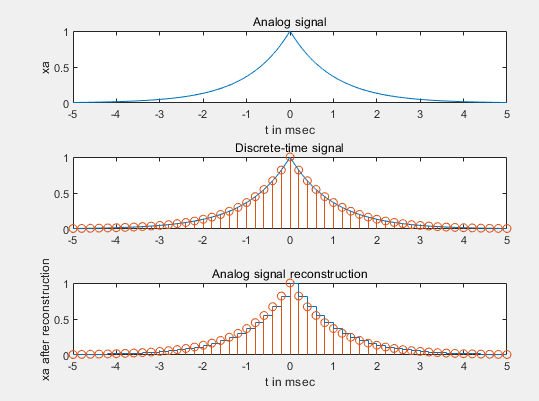第三幅图就是零阶保持恢复信号。

放大第三幅图：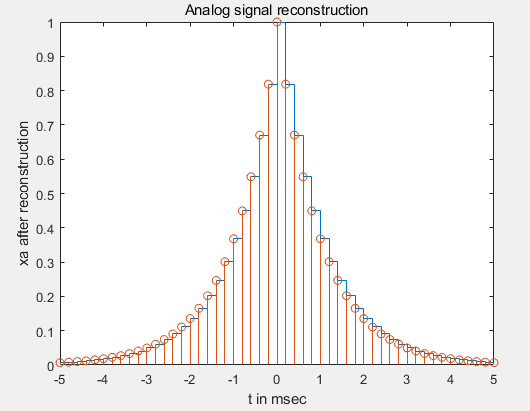当采样率Fs为1000样本/s时，使用零阶保持恢复信号示意图如下：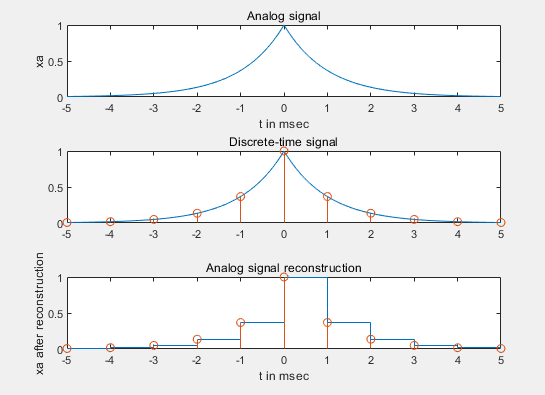放大第三幅图：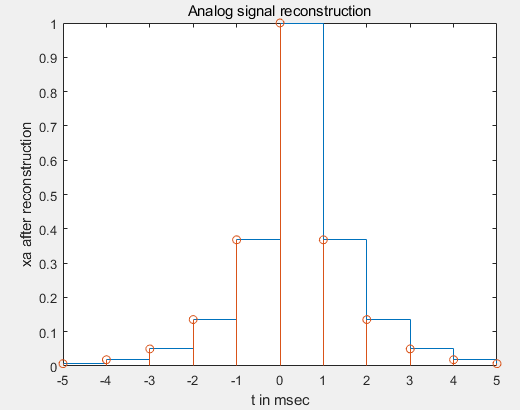零阶保持直接使用stairs函数画出模拟信号的ZOH波形。

可见，采样率为5000时，重建信号比采样率为1000时，重建信号好太多了。但是依然有点粗糙，这就让我们接下来的博文继续探索其他重建方式。


展开全文• 上篇博文采用了零阶保持（ZOH）的方式进行了重构：【 MATLABMATLAB 实现模拟信号采样后的重建（二）零阶保持（ZOH） 这篇博文我们使用一阶保持（FOH）内插来重建信号，采用的案例依然是上篇博文中的案例： 模拟...
上篇博文采用了零阶保持（ZOH）的方式进行了重构：【 MATLAB 】MATLAB 实现模拟信号采样后的重建（二）零阶保持（ZOH）

这篇博文我们使用一阶保持（FOH）内插来重建信号，采用的案例依然是上篇博文中的案例：

模拟信号：

对该信号使用两种不同的采样频率采样。

a. 在 fs = 5000 对信号进行采样

b. 在 fs = 1000 对信号采样

这里直接给出MATLAB脚本：

clc
clear
close all

% Analog signal
Dt = 0.00005;
t = - 0.005:Dt:0.005;
xa = exp(-1000 * abs(t));

subplot(3,1,1);
plot(1000*t,xa);
title('Analog signal');
xlabel('t in msec');
ylabel('xa');

%Fs = 5000,Ts = 0.0002
% Discrete-time signal
Ts = 0.0002;
Fs = 1/Ts;
n = -25:25;
nTs = n*Ts;
x = exp(-1000*abs(nTs));

subplot(3,1,2)
plot(1000*t,xa);
hold on
stem(n*Ts*1000,x);
title('Discrete-time signal');
hold off

% Analog signal reconstruction
subplot(3,1,3);
plot(nTs*1000,x);
title('Analog signal reconstruction');
xlabel('t in msec');
ylabel('xa after reconstruction');
hold on
stem(n*Ts*1000,x)
hold off

% Fs = 1000, Ts = 1ms
% Discrete-time signal
Ts = 0.001;
Fs = 1/Ts;
n = -5:5;
nTs = n*Ts;
x = exp(-1000*abs(nTs));

figure

subplot(3,1,1);
plot(1000*t,xa);
title('Analog signal');
xlabel('t in msec');
ylabel('xa');

subplot(3,1,2)
plot(1000*t,xa);
hold on
stem(n*Ts*1000,x);
title('Discrete-time signal');
hold off

% Analog signal reconstruction
subplot(3,1,3);
plot(nTs*1000,x);
title('Analog signal reconstruction');
xlabel('t in msec');
ylabel('xa after reconstruction');
hold on
stem(n*Ts*1000,x)
hold off



当采样率Fs为5000 样本/s时，用零阶保持的重建信号表示如下：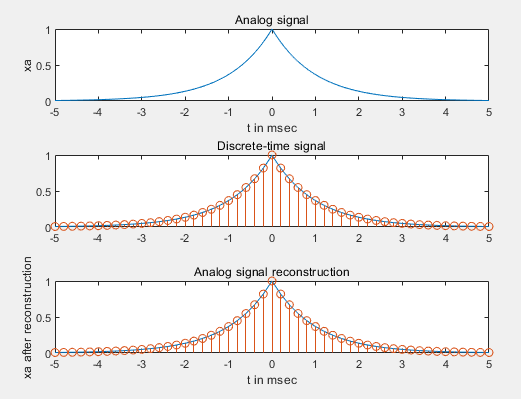第三幅图就是线性内插（FOH）恢复信号。

当采样率Fs为1000样本/s时，使用零阶保持恢复信号示意图如下：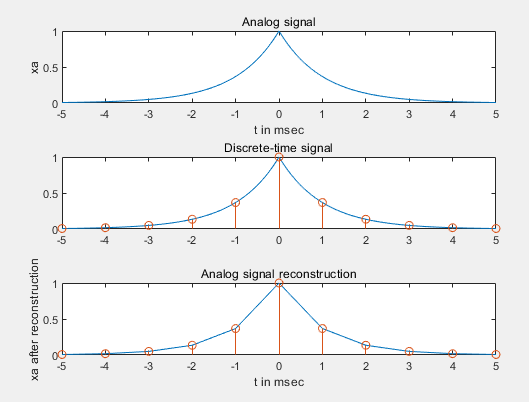第三幅图就是线性内插（FOH）恢复信号。

使用plot函数给出样本之间的线性内插（FOH）。

FOH重建看起来是一种好的重建，一般若采样频率比奈奎斯特频率高很多，那么FOH内插给出一个可以接受的重建。


展开全文• 这篇博文中使用的模拟信号为上篇博文：【 MATLAB 】使用 MATLAB 实现模拟信号的近似及其连续傅里叶变换 ... 在 fs = 1000 对信号采样，求出并画出其离散时间傅里叶变换。 题解： 上篇博文也分析了...
这篇博文中使用的模拟信号为上篇博文：【 MATLAB 】使用 MATLAB 实现模拟信号的近似及其连续傅里叶变换

中使用的模拟信号：

为了研究在频域数量上的采样效果，对该信号使用两种不同的采样频率采样。

a. 在 fs = 5000 对信号进行采样，求出并画出其离散时间傅里叶变换；

b. 在 fs = 1000 对信号采样，求出并画出其离散时间傅里叶变换。

题解：

上篇博文也分析了，信号的带宽为2kHz，奈奎斯特频率就为 4000 样本/s，它小于第一问给出的采样频率，所以频谱混叠几乎不存在。

我们通过MATLAB验证下：

clc
clear
close all

% Analog signal
Dt = 0.00005;
t = - 0.005:Dt:0.005;
xa = exp(-1000 * abs(t));

% Discrete-time signal
Ts = 0.0002;
n = -25:25;
x = exp(-1000*abs(n*Ts));

%Discrete-time Fourier Transform
K = 500;
k = 0:1:K;
w = pi*k/K;
X = x*exp(-j*n'*w);
X = real(X);
w = [-fliplr(w),w(2:K+1)];
X = [fliplr(X),X(2:K+1)];

subplot(2,1,1);
plot(t*1000,xa);
xlabel('t in msec');ylabel('x(n)');
title('Discrete signal');
hold on
stem(n*Ts*1000,x);
gtext('Ts = 0.2msec');
hold off

subplot(2,1,2);
plot(w/pi,X);
xlabel('Frequency in pi units');ylabel('X(w)');
title('Discrete - time Fourier Transform');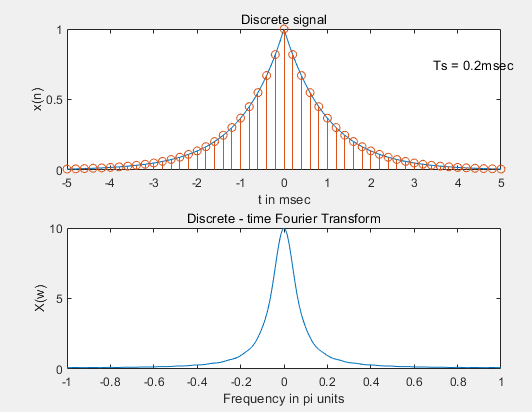继续作答第二问，将采样间隔改为0.001s，10ms时间区间内能采样11个点。

clc
clear
close all

% Analog signal
Dt = 0.00005;
t = - 0.005:Dt:0.005;
xa = exp(-1000 * abs(t));

% Discrete-time signal
Ts = 0.001;
n = -5:5;
x = exp(-1000*abs(n*Ts));

%Discrete-time Fourier Transform
K = 500;
k = 0:1:K;
w = pi*k/K;
X = x*exp(-j*n'*w);
X = real(X);
w = [-fliplr(w),w(2:K+1)];
X = [fliplr(X),X(2:K+1)];

subplot(2,1,1);
plot(t*1000,xa);
xlabel('t in msec');ylabel('x(n)');
title('Discrete signal');
hold on
stem(n*Ts*1000,x);
gtext('Ts = 1msec');
hold off

subplot(2,1,2);
plot(w/pi,X);
xlabel('Frequency in pi units');ylabel('X(w)');
title('Discrete - time Fourier Transform');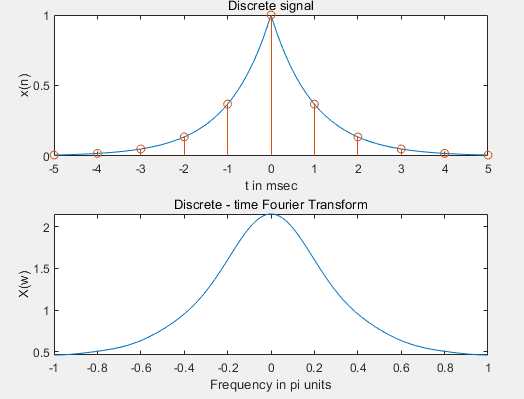很明显，这个DTFT7已经变了，也就是发生了频谱混叠。
展开全文• 前三篇博文讲了三种方法进行内插重建信号： sinc函数内插重建 零阶保持（ZOH）内插重建 一阶保持内插（FOH）重建 这篇文章使用三次样条函数spline来实现内插重建，并... 在 fs = 1000 对信号采样 前面太多的文...
前三篇博文讲了三种方法进行内插重建信号：

sinc函数内插重建

零阶保持（ZOH）内插重建

一阶保持内插（FOH）重建

这篇文章使用三次样条函数spline来实现内插重建，并分析重建误差。

采用的案例依然是上篇博文中的案例：

模拟信号：

对该信号使用两种不同的采样频率采样。

a. 在 fs = 5000 对信号进行采样

b. 在 fs = 1000 对信号采样

前面太多的文章作为铺垫，这里直接给出MATLAB脚本：

clc
clear
close all

% Analog signal
Dt = 0.00005;
t = - 0.005:Dt:0.005;
xa = exp(-1000 * abs(t));

subplot(3,1,1);
plot(1000*t,xa);
title('Analog signal');
xlabel('t in msec');
ylabel('xa');

%Fs = 5000,Ts = 0.0002
% Discrete-time signal
Ts = 0.0002;
Fs = 1/Ts;
n = -25:25;
nTs = n*Ts;
x = exp(-1000*abs(nTs));

subplot(3,1,2)
plot(1000*t,xa);
hold on
stem(n*Ts*1000,x);
title('Discrete-time signal');
hold off

% Analog signal reconstruction
subplot(3,1,3);
xa_r = spline(nTs,x,t);
plot(t*1000,xa_r);
title('Analog signal reconstruction');
xlabel('t in msec');
ylabel('xa after reconstruction');
hold on
stem(n*Ts*1000,x)
hold off

error1 = max(abs(xa_r - xa))

% Fs = 1000, Ts = 1ms
% Discrete-time signal
Ts = 0.001;
Fs = 1/Ts;
n = -5:5;
nTs = n*Ts;
x = exp(-1000*abs(nTs));

figure

subplot(3,1,1);
plot(1000*t,xa);
title('Analog signal');
xlabel('t in msec');
ylabel('xa');

subplot(3,1,2)
plot(1000*t,xa);
hold on
stem(n*Ts*1000,x);
title('Discrete-time signal');
hold off

% Analog signal reconstruction
subplot(3,1,3);
xa_r = spline(nTs,x,t);
plot(1000*t,xa_r);
title('Analog signal reconstruction');
xlabel('t in msec');
ylabel('xa after reconstruction');
hold on
stem(n*Ts*1000,x)
hold off

error2 = max(abs(xa_r - xa))



当采样率Fs为5000 样本/s时，重建结果：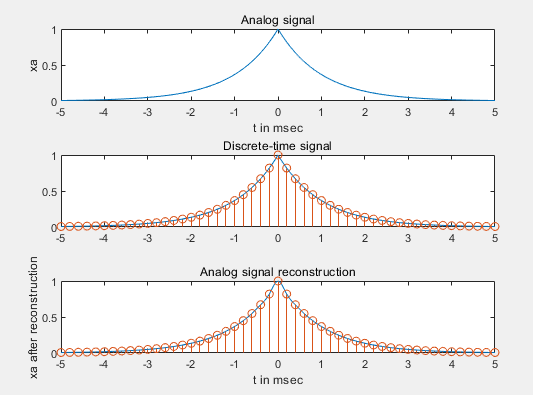误差：

error1 =

0.0317

重建和真正的模拟信号之间的误差是0.0317，这是由于非理想内插和非带限造成的。将这个误差与sinc函数内插比较（理想），这个误差还比较小。从重构图上看，重建还是不错的。

当采样率Fs为1000样本/s时，重构结果：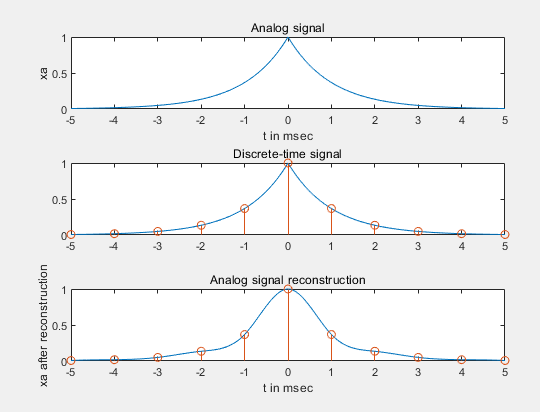error2 =

0.1679

这个情况下误差较大，这是由于采样间隔过大，也就是采样率过低造成的频谱混叠造成的，不能恢复原始信号了。


展开全文• 一、在matlab中如何表示频率为f1，以采样率f抽样后所得到的数字信号？如此表示的依据是什么？ 二、使用matlab画出的频谱(一般是幅度谱或称振幅谱)的横坐标轴的意义是什么？如何根据横坐标轴的值得到其所对应的实际...
• 前提：根据采样定理，心率信号采样频率为50hz （大于心率的频率两倍），得带数据信号数据源“B.TXT”文件。 1. 原始数据的采集截取有效部分分析。 2.数据滤波（50Hz的工频干扰以及放大器本身的漂移），要根据频谱...
• b站视频地址：...方法一：直接使用数字滤波器，采样频率至少是最高频率的两倍，为了不让高频成分发生频谱混叠，这时采样频率必须非常高 方法二：如果先经过一个模拟滤波器，将高频
• b=menu('请选择：','原始信号采样后的时域图和频谱图','FIR滤波器','IIR滤波器','退出'); if b==1 for j=1:3 temp=menu('请选择','播放原始语音','原始语音时域图和频谱图','FFT频谱图'); if temp==1 ...
• 在做通信系统仿真时，我们常常需要画出信号的频谱图来进行信号的分析，但是MATLAB中只提供了 fft 函数，因此每次总要写出几条语句来画出信号的频谱，十分不方便。所以我自己写了一个频谱绘制函数 SpecPlot ，可以...数字通信
• ex07150b 频率采样技术: 低通，最优法 ex071600 频率采样技术: 低通，最优法 T1和T2 ex071700 频率采样技术: 带通，最优法 T1 和 T2 ex071800 频率采样技术: 高通，最优法 T1和T2 ex071900 频率采样技术: 差分器 ex...m文件
• Chirp信号的表达式如下： x(t)=exp(j2π(f0t+12kt2))x(t)=exp(j2\pi (f_0t+\frac{1}{2} kt^2))x(t)=exp(j2π(f0​...给定信号信号时间长度为50ms 带宽 B=5KHz,初始频率f0=3KHzf_0=3KHzf0​=3KHz 采样频率为 fsf_sfs​ =
• 一、在matlab中如何表示频率为f1，以采样率f抽样后所得到的数字信号？如此表示的依据是什么？ 二、使用matlab画出的频谱(一般是幅度谱或称振幅谱)的横坐标轴的意义是什么？如何根据横坐标轴的值得到其所对应的...
• 用振幅为0.8的方波进行Fourier分析，并用分析得到的系数求解当k取不同值时的合成信号 程序如下; clear all; close all;%关闭所有打开的所有图形窗口 N=200;dt=4/N;%数据点数和采样间隔...plot input c
• %对信号进行快速Fourier变换 mag=abs(y); n=0:N-1;%求得Fourier变换后的振幅 f=n*fs/N; %频率序列 figure(1); subplot(2,1,1),plot(f,mag); xlabel('频率(Hz)');title('原声音幅频响应'); %绘出随频率变化的振幅 ...
• 在一次课设中使用到了匹配滤波器进行LFM信号处理，所以打算记下来，如果有错误，希望评论区指出。时间（12月21日） clear all,clc; %基本变量的申请 fs = 2000 * 100000; %采样的频率 Ts = 1/fs; %采样周期 N = 2000...
• 录制一段自己的语音信号，并对录制的信号进行采样；画出采样后语音信号的时域波形和频谱图；给定滤波器的性能指标，采用窗函数法和双线性变换设计滤波器，并画出滤波器的频率响应；然后用自己设计的滤波器对采集的...
• 主函数中采用8个天线，产生k=128个周期的线性调频信号，该信号的频率为77GHz，调制周期为38.9e-6 s ,调制带宽B为350MHz，并对产生的每一个周期的信号进行了N=256点采样。在产生的信号中设置的目标数为4，这4个目标的...
• 采用定步长算法，在当你的信号频率大于求解器的频率的时候，你就可能丢失掉一些点，因而是危险的。采用变步长算法可以确保在低频的时候采用大的步长，而在高频的时候采用小的步长。MATLAB中的ODE求解器优化了变步长...
• % 对I进行采样，得到与信源序列A'相同样点数量的恢复信号序列（J）； % 对J与A'进行比较，完成误码率判决，并绘制误码率曲线； ———————————————— 版权声明：本文为CSDN博主「qq_29249849」的...
• 以整数sps（插入零）的系数对矩阵xin中的输入数据进行采样b.使用cconv用矢量或矩阵编号中给出的脉冲响应序列过滤上采样信号数据。 假设信号正在循环； c.恢复过滤器延迟。 function [txSymsDMAuthentic , ...
• 语音信号是瞬时变化的，但在10～20ms内是相对稳定的，而我们设定的采样频率为11025所以我们对预处理后的语音信号S1(n)以300点为一帧进行处理，帧移为100个采样点。 （N=300） D 窗化处理： 为了避免矩形窗化时对...
• 原文：...对于一个时域信号x(t)，采样频率为Fs,信号频率F,采样点数为N，进行N采样后为y[n],则fft(y,N)计算所得的结果就是一个为N点的复数a+bi. 每一个点就对应着一个频率点.这个点的模值,就是...FFT
• 原文：...对于一个时域信号x(t)，采样频率为Fs,信号频率F,采样点数为N，进行N采样后为y[n],则fft(y,N)计算所得的结果就是一个为N点的复数a+bi. 每一个点就对应着一个频率点.这个点的模值,就是...
• 基于VC_与Matlab的数字信号处理实验平台设计.pdf 基于VC_与OpenGL的飞秒激光加工几何仿真系统的研究.pdf 基于VC_与PMAC的机器人控制软件的开发.pdf 基于VC_串口通信.pdf 基于VC_和COM的复杂系统控制算法的研究.pdf ...
• for j=1:(total) % 串行第一路信号采样 if xulie(k)==1 NRZ_0((k-1)*total+j)=1; nrz_0(k)=1; else NRZ_0((k-1)*total+j)=-1; nrz_0(k)=-1; end end for j=1:(total) % 串行第二路信号采样 if ...开发语言 开发语言
• https://wenku.baidu.com/view/9eacc76bb84ae45c3b358cba.html 此网址可让fft变换中相关的参数讲解的...假设采样频率为Fs，信号频率F，采样点数为N。那么FFT之后结果就是一个为N点的复数。每一个点就对应着一个频率...
• 基于VC_与Matlab的数字信号处理实验平台设计.pdf 基于VC_与OpenGL的飞秒激光加工几何仿真系统的研究.pdf 基于VC_与PMAC的机器人控制软件的开发.pdf 基于VC_串口通信.pdf 基于VC_和COM的复杂系统控制算法的研究.pdf ...# matlabb进行信号采样matlab 订阅